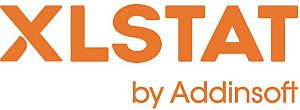# XLSTAT - Non-linear regression

## When to use nonlinear regression

Nonlinear regression is used to model complex phenomena which cannot be handled by the linear model. XLSTAT provides preprogrammed functions from which the user may be able to select the model which describes the phenomenon to be modeled.

## Options for Nonlinear regression in XLSTAT

When the model required is not available, the users can define a new model and add it to their personal library. Microsoft Excel functions can be used: Exp(), Sin(), Pi(), Max(), etc.

When this is possible the Levenberg-Marquardt algorithm is used. When the derivatives are not available, a more complex and slower but efficient algorithm is used.

## Results for nonlinear regression in XLSTAT

• Correlation matrix:
This table displays the correlations between the selected variables.
• Goodness of fit coefficients:
This table shows the following statistics:
• The number of observations;
• The degrees of freedom (DF);
• The determination coefficient R2;
• The sum of squares of the errors (or residuals) of the model (SSE or SSR respectively);
• The means of the squares of the errors (or residuals) of the model (MSE or MSR);
• The root mean squares of the errors (or residuals) of the model (RMSE or RMSR);
• Model parameters:
This table gives the value of each parameter after fitting to the model. For built-in functions, or user-defined functions when derivatives for the parameters have been entered, the standard deviations of the estimators are calculated.
• Predictions and residuals:
This table gives for each observation the input data, the value predicted by the model and the residuals. It is followed by the equation of the model.

## Charts for nonlinear regression in XLSTAT

If only one quantitative explanatory variable has been selected, the first chart represents the data and the curve for the chosen function. The second chart is the bar chart of the residuals.This analysis is available in the XLStat-Basic addin for Microsoft ExcelKovach Computing Services (KCS) was founded in 1993 by Dr. Warren Kovach. The company specializes in the development and marketing of inexpensive and easy-to-use statistical software for scientists, as well as in data analysis consulting.

### Get in Touch

• Email:
sales@kovcomp.com